x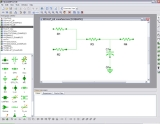EcosimEncyclopedia
EcosimPro is a simulation tool developed by Empresarios Agrupados A.I.E for modelling simple and complex physical processes that can be expressed in terms of Differential algebraic equations or Ordinary differential equations and Discrete event simulation
Discrete Event Simulation
In discrete-event simulation, the operation of a system is represented as a chronological sequence of events. Each event occurs at an instant in time and marks a change of state in the system...

.

The application runs on the various Microsoft Windows
Microsoft Windows
Microsoft Windows is a series of operating systems produced by Microsoft.Microsoft introduced an operating environment named Windows on November 20, 1985 as an add-on to MS-DOS in response to the growing interest in graphical user interfaces . Microsoft Windows came to dominate the world's personal...

platforms and uses its own graphic environment for model design.

The modelling of physical components is based on the EcosimPro language (EL) which is very similar to other conventional Object-oriented programming
Object-oriented programming
Object-oriented programming is a programming paradigm using "objects" – data structures consisting of data fields and methods together with their interactions – to design applications and computer programs. Programming techniques may include features such as data abstraction,...

languages but is powerful enough to model continuous and discrete processes.

This tool employs a set of libraries containing various types of components (mechanical, electrical, pneumatic, hydraulic, etc.) that can be reused to model any type of system.

It is used within ESA for propulsion systems analysis and is the recommended ESA analysis tool for ECLS systems.

## Origins

The EcosimPro Tool Project began in 1989 with funds from the European Space Agency
European Space Agency
The European Space Agency , established in 1975, is an intergovernmental organisation dedicated to the exploration of space, currently with 18 member states...

(ESA) and with the goal of simulating environmental control and life support systems for manned spacecraft, such as the Hermes shuttle
Hermes (shuttle)
Hermes was a proposed spaceplane designed by the French Centre National d'Études Spatiales in 1975, and later by the European Space Agency. It was superficially similar to the US X-20. France proposed in January 1985 to go through with Hermes development under the auspices of the ESA. Hermes was...

.
The multidisciplinary nature of this modelling tool led to its use in many other disciplines, including fluid mechanics, chemical processing, control, energy, propulsion and flight dynamics. These complex applications have demonstrated that EcosimPro is very robust and ready for use in many other fields.

### Code examples

Differential equation

To familiarize yourself with the use of EcosimPro, first create a simple component to solve a differential equation. Although EcosimPro is designed to simulate complex systems, it can also be used independently of a physical system as if it were a pure equation solver. The example in this section illustrates this type of use. It solves the following differential equation to introduce a delay to variable x: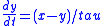which is equivalent to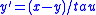where x and y have a time dependence that will be defined in the experiment. Tau is datum provided given by the user; we will use a value of 0.6 seconds. This equation introduces a delay in the x variable with respect to y with value tau. To simulate this equation we will create an EcosimPro component with the equation in it.

The component to be simulated in EL is like thus:

```COMPONENT equation_test
DATA
REAL tau = 0.6      "delay time (seconds)"
DECLS
REAL x, y
CONTINUOUS
y' = (x - y) / tau
END COMPONENT```

Pendulum

One example of applied calculus could be the movement of a perfect pendulum (no friction taken into account). We would have the following data: the force of gravity ‘g’; the length of the pendulum ‘L’; and the pendulum’s mass ‘M’. As variables to be calculated we would have: the Cartesian position at each moment in time of the pendulum ‘x’ and ‘y’ and the tension on the wire of the pendulum ‘T’. The equations that define the model would be:

- Projecting the length of the cable on the Cartesian axes and applying Pythagoras’ theorem we get: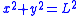By decomposing force in Cartesians we get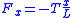and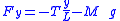To obtain the differential equations we can convert: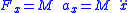and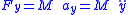(note: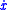is the first derivative of the position and equals the speed.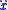is the second derivative of the position and equals the acceleration)

This example can be found in the DEFAULT_LIB library as “pendulum.el”: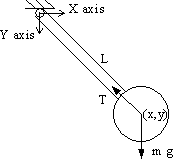```COMPONENT pendulum   "Pendulum example"
DATA
REAL g = 9.806               "Gravity (m/s^2)"
REAL L = 1.                  "Pendulum longitude (m)"
REAL M = 1.                  "Pendulum mass (kg)"
DECLS
REAL x                       "Pendulum X position (m)"
REAL y                       "Pendulum Y position (m)"
REAL T                       "Pendulum wire tension force (N)"
CONTINUOUS
x**2 + y**2 = L**2
M * x = - T * (x / L)
M * y = - T * (y / L) - M * g
END COMPONENT```

The last two equations respectively express the accelerations, x’’ and y’’, on the X and Y axes

## Maths capabilities

• Symbolic handling of equations (e.g.: derivation, etc.)
• Robust solvers for non-linear and DAE systems: DASSL, Newton-Raphson

• Math wizards for:
• Defining boundary conditions
• Solving algebraic loops
• Reducing high-index DAE problems
• Clever mathematical algorithms based on graph theory to minimize the number of unknown variables and equations
• Powerful discrete events handler to stop simulation when an event occurs

## Applications

EcosimPro has been used in many fields and disciplines. The following paragraphs show several applications
• Control: This library provides components for the representation of control loops, including the typical P, PI and PID controllers, and signal processors, etc.
• Turbojet: Library for modelling turbine reactors. With components such as turbines, nozzles, compressors, burners, etc.
• ECLSS: A complete library of components has been developed to model complex environmental conditions for manned spacecraft
• ESPSS: A standard set of libraries with components and functions for the simulation of launch vehicle propulsion systems and spacecraft propulsion systems.
• Thermal: This library contains the components necessary to develop Lumped Parameter Thermal Models, i.e., diffusive thermal nodes, boundary thermal nodes, linear thermal conductors and radiative thermal conductors.
• Energy: In the field of Energy, EcosimPro has been used in different applications such as heat balances (Thermal_Balance), hydraulic systems (Pipe Networks Tool), molten carbonate and alkaline fuel cells, etc.
• Cryogenics: Simulation of large cryogenics systems, for instace, at CERN
CERN
The European Organization for Nuclear Research , known as CERN , is an international organization whose purpose is to operate the world's largest particle physics laboratory, which is situated in the northwest suburbs of Geneva on the Franco–Swiss border...

.
• Others:
• Water treatment
• Waste treatment
• Agri-food Biotech processes
• Etc.